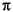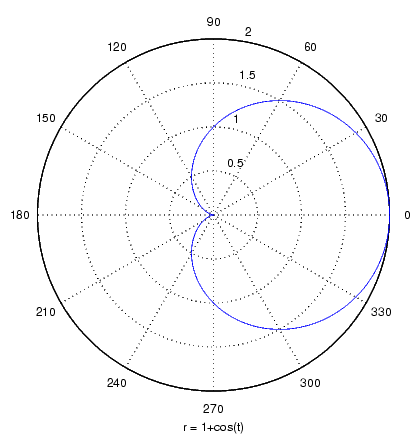MATLAB Function Referenceezpolar

Easy to use polar coordinate plotter

Syntax

• ```ezpolar(fun)
ezpolar(fun,[a,b])
ezpolar(axes_handle,...)
h = ezpolar(...)
```

Description

```ezpolar(fun) ``` plots the polar curve `rho = fun(theta)` over the default domain 0 < `theta` < 2.

`fun` can be a function handle for an M-file function or an anonymous function (see Function Handles and Anonymous Functions) or a string (see the Remarks section).

```ezpolar(fun,[a,b]) ``` plots `fun` for `a` < `theta` < `b`.

```ezpolar(axes_handle,...) ``` plots into the axes with handle `axes_handle` instead of the current axes (`gca`).

```h = ezpolar(...) ``` returns the handle to a line object in `h`.

Remarks

Passing the Function as a String

Array multiplication, division, and exponentiation are always implied in the expression you pass to `ezpolar`. For example, the MATLAB syntax for a plot of the expression

• ```t.^2.*cos(t)
```

which represents an implicitly defined function, is written as

• ```ezpolar('t^2*cos(t)')
```

That is, `t^2` is interpreted as `t.^2` in the string you pass to `ezpolar`.

Passing a Function Handle

Function handle arguments must point to functions that use MATLAB syntax. For example, the following statements define an anonymous function and pass the function handle `fh` to `ezpolar`.

• ```fh = @(t) t.^2.*cos(t);
ezpolar(fh)
```

Note that when using function handles, you must use the array power, array multiplication, and array division operators (`.^, .*, ./`) since `ezpolar` does not alter the syntax, as in the case with string inputs.

Passing Additional Arguments

If your function has additional parameters, for example `k1` and `k2` in `myfun`:

• ```function s = myfun(t,k1,k2)
s = sin(k1*t).*cos(k2*t);
```

then you can use an anonymous function to specify the parameters:

• ```ezpolar(@(t)myfun(t,2,3))
```

Examples

This example creates a polar plot of the function

1 + cos(t)

over the domain [0, 2]:

• ```ezpolar('1+cos(t)')```

See Also

`ezplot`, `ezplot3`, `function_handle`, `plot`, `plot3`, `polar`

Function Plots for related functions

© 1994-2005 The MathWorks, Inc.